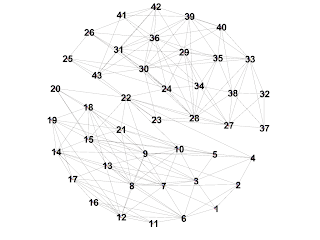## Thursday, March 08, 2012

### Truss Extraction Algorithm in MapReduce&HBase

In this post I will cover implementation of the Truss Extraction Algorithm (TEA) , adapted to be used in Hadoop MapReduce&HBase environment.

First of all, let us cite Jonathan Cohen's work to clarify what we are actually after:

A k-truss is a non-trivial, one-component subgraph such that each edge is
reinforced by at least k–2 pairs of edges making a triangle with the that edge. (Non-
trivial here excludes an isolated vertex as a truss.)

Fundamental question we have to answer before getting drowned in bits and bytes - what is so valuable in truss? How can we benefit from having ability to associate network player with one group or another? As proverb goes "Tell me your friends, and I'll tell you who you are".

Since we have mentioned graphs, let us also provide some illustration:Figure 1: Typical example of social graph, where players present nodes, and edges - social connections.Figure 2: Trusses extracted from example of Figure 1. In this case support threshold was set to 4.
Discussed algorithm is adaptation of Cohen's Truss Extraction Algorithm in MapReduce environment. Main improvement I bring in here is usage of HBase for graph definition, holding working structures and final truss graphs.

Overall, there are 6 steps in the algorithm.

Step 0: Graph simplification
This is business-sensitive step, which presents functionality outside of TEA body, and thus - will be presented in high-level details.

Input: social data (i.e. number of private messages, number of comments, user's contact list, etc) for a relationship between user_a and user_b.
Each positive fact should result in positive value, and undesired - in negative.
Further - we sum all values for given relationship and if sum crosses some predefined threshold, we consider this relationship as worth processing.

Output: table TABLE_TRUSS_TEMP in format:
 family user row user_a user_b user_c user_d user_a d(A), d(B) d(A), d(C) d(A), d(D) user_b d(B), d(A) d(B), d(C) d(B), d(D) user_c d(C), d(A) d(C), d(B) d(C), d(D) user_d d(D), d(A) d(D), d(B) d(D), d(C)

where d(X) presents valency of the node X.

Step 1: Updating valencies in the table TABLE_TRUSS_TEMP
Steps 1-2-3-4 are executed in loop. Step 1 shall be skipped for the first time, since TABLE_TRUSS_TEMP is in its initial state left by Step 0.

***mapper***
reads table in format:
key: UserA    value for column B: d(A), d(B)
key: UserB    value for column A: d(B), d(A)

output in format:
key: (A, B)    value: A, d(A)
key: (A, B)    value: B, d(B)
where A < B by numerical comparison

***reducer***
receives input in format:
key: (A, B)    value: A, d(A)
key: (A, B)    value: B, d(B)
where A < B by numerical comparison

output format:
key: UserA    value for column B: d(A), d(B)
key: UserB    value for column A: d(B), d(A)

Step 2: First phase of triangle enumeration
Truss extraction algorithm is build on top of triangle enumeration algorithm . In this and next steps we calculate number of triangles for every edge in the graph.
In social networks this has the same meaning as number of mutual friends for any relationship.

***mapper***
reads TABLE_TRUSS_TEMP in format:
key: UserA    value for column B: d(A), d(B)
key: UserB    value for column A: d(B), d(A)
and emits output in format:
key: vertex V    value: edge (V', V''), d(V'), d(V'')
where V := d(V') < d(V'') ? V' : (|V'| < |V''| ? V' : V'');
in case d(V') == d(V''), V' < V'' by numerical comparison

***reducer***
input:
key: vertex A    value: [[(A, B), d(A), d(B)], [(A, C), d(A), d(C)] ...]
where:
d(A) < d(B) or if d(A) == d(B) : A < B by numerical comparison
d(A) < d(C) or if d(A) == d(C) : A < C by numerical comparison

outputs to file "step2.out":
key: edge (B, C)    value (A, B), (A, C)
key: edge (B, C)    value (B, C)
where d(B) < d(C)
in case d(B) == d(C), B < C by numerical comparison

Step 3: Second phase of triangle enumeration
***mapper***
Identity mapper with input from "step2.out"

***reducer***
input in format:
key: edge (B, C)    value (A, B), (A, C)
key: edge (B, C)    value (B, C)

outputs to file "step3.out":
emit each edge for every triangle it is in
so, in summary we have:
key: edge (A, B)    value: 2 (number of triangles AB is in)

For every edge in open pair or every solitary edge, we are emitting ZERO:
key: edge (K, L)    value: 0 (ZERO)

Step 4: Dropping edges with insufficient support
As soon as we have tuple of [edge & number of triangles it is in], we can define if edge support is sufficient; otherwise the edge is dropped.

***mapper***
Identity mapper with input from "step3.out"

***reducer***
input example:
key: edge (A, B)    value: 2 (number of triangles AB is in)
key: edge (C, D)    value: 5 (number of triangles CD is in)

output:
emits Delete for every edge that has _insufficient_ support (|edge| <= threshold)

Workflow: Looping condition
Step1-Step2-Step3-Step4 are repeated in loop until number of dropped edges is 0

Step 5: Truss Identification Algorithm (TIA)
At this point, we have dropped all edges from TABLE_TRUSS_TEMP that are not part of trusses. TIA is 3-step procedure and goes beyond topic of this post.
However, we can assume that at the end of the TIA we get table TABLE_TRUSS in format:

 family user row user_a user_b user_c user_d Truss_id_1 1 1 1 1

where Truss_id_1 is unique Truss identification, that allows insignificant changes to the truss structure (for instance: truss has been left or joined by non-prominent player).

Performance:
For a social graph with:
• 64.5K nodes
• number of edges varying from 1 to 15723 per node
and environment:
• AWS hosting
• 5 m1.large instances (8GB RAM, 2 Cores)
Algorithm completes in 25 iterations, that take per average 1 Day 7 Hours.

 Truss extraction algorithm

 Truss Extraction algorithm in MapReduce

 Triangle enumeration